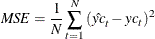The MODEL Procedure

Solution Mode Output

Subsections:

The following SAS statements dynamically forecast the solution to a nonlinear equation:

proc model data=sashelp.citimon;
parameters a 0.010708  b  -0.478849 c 0.929304;
lhur = 1/(a * ip) + b + c * lag(lhur);
solve lhur / out=sim forecast dynamic;
run;


The first page of output produced by the SOLVE step is shown in Figure 19.77. This is the summary description of the model. The error message states that the simulation was aborted at observation 144 because of missing input values.

Figure 19.77: Solve Step Summary Output

The MODEL Procedure

Model Summary
Model Variables 1
Parameters 3
Equations 1
Number of Statements 1
Program Lag Length 1

Model Variables LHUR a(0.010708) b(-0.478849) c(0.929304) LHUR

The second page of output, shown in Figure 19.78, gives more information on the failed observation.

Figure 19.78: Solve Step Error Message

The MODEL Procedure
Dynamic Single-Equation Forecast

 Solution values are missing because of missing input values for observation 144 at NEWTON iteration 0.

 Note: Additional information on the values of the variables at this observation, which may be helpful in determining the cause of the failure of the solution process, is printed below.

Observation Iteration CC 144 0 -1.000000 1

 Iteration Errors - Missing.

  The MODEL Procedure Dynamic Single-Equation Forecast --- Listing of Program Data Vector --- _N_: 144 ACTUAL.LHUR: . ERROR.LHUR: . IP: . LHUR: 7.10000 PRED.LHUR: . a: 0.01071 b: -0.47885 c: 0.92930 

 Note: Simulation aborted.

From the program data vector, you can see the variable IP is missing for observation 144. LHUR could not be computed, so the simulation aborted.

The solution summary table is shown in Figure 19.79.

Figure 19.79: Solution Summary Report

The MODEL Procedure
Dynamic Single-Equation Forecast

Data Set Options
DATA= SASHELP.CITIMON
OUT= SIM

Solution Summary
Variables Solved 1
Forecast Lag Length 1
Solution Method NEWTON
CONVERGE= 1E-8
Maximum CC 0
Maximum Iterations 1
Total Iterations 143
Average Iterations 1

Observations Processed
Lagged 1
Solved 143
First 2
Last 145
Failed 1

Variables Solved For LHUR

This solution summary table includes the names of the input data set and the output data set followed by a description of the model. The table also indicates that the solution method defaulted to Newton’s method. The remaining output is defined as follows.

 Maximum CC is the maximum convergence value accepted by the Newton procedure. This number is always less than the value for the CONVERGE= option. Maximum Iterations is the maximum number of Newton iterations performed at each observation and each replication of Monte Carlo simulations. Total Iterations is the sum of the number of iterations required for each observation and each Monte Carlo simulation. Average Iterations is the average number of Newton iterations required to solve the system at each step. Solved is the number of observations used times the number of random replications selected plus one, for Monte Carlo simulations. The one additional simulation is the original unperturbed solution. For simulations that do not involve Monte Carlo, this number is the number of observations used.

Summary Statistics

The STATS and THEIL options are used to select goodness-of-fit statistics. Actual values must be provided in the input data set for these statistics to be printed. When the RANDOM= option is specified, the statistics do not include the unperturbed (_REP_=0) solution.

STATS Option Output

The following statements show the addition of the STATS and THEIL options to the model in the previous section:

proc model data=sashelp.citimon;
parameters a 0.010708  b  -0.478849 c 0.929304;
lhur= 1/(a * ip) + b + c * lag(lhur) ;
solve lhur / out=sim dynamic stats theil;
range date to '01nov91'd;
run;


The STATS output in Figure 19.80 and the THEIL output in Figure 19.81 are generated.

Figure 19.80: STATS Output

The MODEL Procedure
Dynamic Single-Equation Simulation

Solution Range DATE = FEB1980 To NOV1991

Descriptive Statistics
Variable N Obs N Actual Predicted Label
Mean Std Dev Mean Std Dev
LHUR 142 142 7.0887 1.4509 7.2473 1.1465 UNEMPLOYMENT RATE: ALL WORKERS, 16 YEARS

Statistics of fit
Variable N Mean Error Mean % Error Mean Abs Error Mean Abs % Error RMS Error RMS % Error R-Square Label
LHUR 142 0.1585 3.5289 0.6937 10.0001 0.7854 11.2452 0.7049 UNEMPLOYMENT RATE: ALL WORKERS, 16 YEARS

The number of observations (Nobs), the number of observations with both predicted and actual values nonmissing (N), and the mean and standard deviation of the actual and predicted values of the determined variables are printed first. The next set of columns in the output are defined as follows:

 Mean Error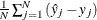Mean % Error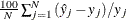Mean Abs Error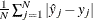Mean Abs % Error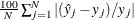RMS Error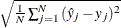RMS % Error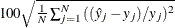R-square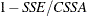SSE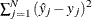SSA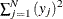CSSA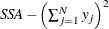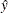predicted value y actual value

When the RANDOM= option is specified, the statistics do not include the unperturbed (_REP_=0) solution.

THEIL Option Output

The THEIL option specifies that Theil forecast error statistics be computed for the actual and predicted values and for the relative changes from lagged values. Mathematically, the quantities are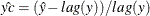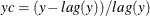where
is the relative change for the predicted value and
is the relative change for the actual value.

Figure 19.81: THEIL Output

Theil Forecast Error Statistics
Variable N MSE Corr (R) MSE Decomposition Proportions Inequality Coef Label
Bias (UM) Reg (UR) Dist (UD) Var (US) Covar (UC) U1 U
LHUR 142 0.6168 0.85 0.04 0.01 0.95 0.15 0.81 0.1086 0.0539 UNEMPLOYMENT RATE: ALL WORKERS, 16 YEARS

Theil Relative Change Forecast Error Statistics
Variable Relative Change MSE Decomposition Proportions Inequality Coef Label
N MSE Corr (R) Bias (UM) Reg (UR) Dist (UD) Var (US) Covar (UC) U1 U
LHUR 142 0.0126 -0.08 0.09 0.85 0.06 0.43 0.47 4.1226 0.8348 UNEMPLOYMENT RATE: ALL WORKERS, 16 YEARS

The columns have the following meaning:

Corr (R)

is the correlation coefficient,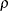, between the actual and predicted values.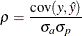where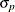and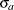are the standard deviations of the predicted and actual values.

Bias (UM)

is an indication of systematic error and measures the extent to which the average values of the actual and predicted deviate from each other.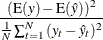Reg (UR)

is defined as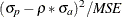. Consider the regression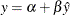If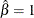, UR will equal zero.

Dist (UD)

is defined as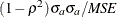and represents the variance of the residuals obtained by regressingon.

Var (US)

is the variance proportion. US indicates the ability of the model to replicate the degree of variability in the endogenous variable.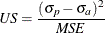Covar (UC)

represents the remaining error after deviations from average values and average variabilities have been accounted for.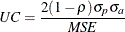U1

is a statistic that measures the accuracy of a forecast defined as follows: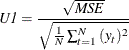U

is the Theil’s inequality coefficient defined as follows:MSE

is the mean square error. In the case of the relative change Theil statistics, the MSE is computed as follows: